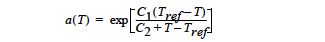# Polymer Shift Function#

```Polymer Shift Function = {CONSTANT | MODIFIED_WLF} <float1> [float2]
```

## Description / Usage#

This optional card is used to specify the temperature shift function for the polymer relaxation times and viscosities in the polymer stress equation(s);

Valid options are

 CONSTANT Applies a constant temperature shift factor to the polymer relaxation time(s) and the polymer viscosities. - the temperature shift factor. If this card is not present, this option is the default and a shift factor of 1.0 is applied.

This option may be useful for continuation in elasticity level since continuation in this parameter will uniformly increase or decrease the relaxation time(s) and viscosities of all viscoelastic modes.

 MODIFIED_WLF Applies a temperature shift factor which is a modified version of the Williams-Landel-Ferry shift model (cf. Bird, Armstrong, and Hassager 1987, pp.139-143);The reference temperature, Tref, is taken from the Reference Temperature card. Note that if C2 is chosen equal to Tref, this model reduces to an Arrhenius form where C1 = Eμ/RTref. Also note that this form is based on the exponential function whereas the WLF model is based on 10x. - constant C1 - constant C2

## Examples#

The following is a sample card that sets a constant temperature shift.

```Polymer Shift Function = CONSTANT 1.0
```

The following is a sample card that utilizes the modified WLF shift function.

```Polymer Shift Function = MODIFIED_WLF 2.5 95.0
```

No Discussion.